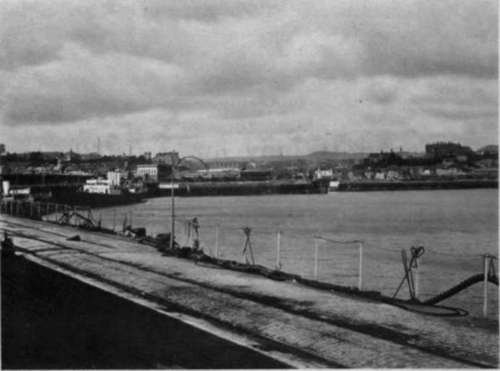By CAPT. OWEN WHEELER, F.R.P.S.

If you take an ordinary photographic lens and place behind it, at a suitable separation, a negative lens (i.e., one which diminishes objects viewed through it), you produce - assuming that the negative lens is of less focal length than the ordinary or " positive " lens - a sort of photographic telescope. In other words, you enable the image of an object, which the ordinary photographic lens renders quite small, to be magnified, the degree of enlargement depending upon (1) the relation of the focal length of the negative lens to the focal length of the positive lens, (2) the separation between the positive and negative elements, and (3) the camera extension. That, in a nutshell, is Telephotography and the optical combination produced in this way is a telephoto lens.

As regards (1) it is sufficient here to say that, theoretically, you can combine any negative lens with any positive lens so long as the latter has a greater focal length than the former. The greater the disparity the greater the " power " or degree of magnification with a certain camera extension. Thus, if we have a 6-in. positive, and a 12-in. camera extension, we get a higher magnification with a 3-in. negative than with a 4-in.Capt. Owen Wheeler, F.R.P.S.Folkestone Harbour.

From two negatives taken from the same spot without moving the camera. By Captain Owen Wheeler, F.R.P.S.

The separation between the two elements (2) is a subject which even the beginner should take pains to master. For telephotographic purposes it lies between two extremes, one being the focal length of the positive, the other the difference of the focal lengths of the positive and negative. If we have a 6-in. positive and a 2-in. negative the separation - measured from the nodes of the two elements - will vary between a fraction less than 6 in. and a fraction more than 6 in. - 2 in. = 4 in. If it is 6 in. there is no magnification ; if it is 4 in. the combination ceases to be a telephoto lens and becomes an ordinary telescope. Between the two extremes the magnification increases as the separation decreases, until theoretically the degree of enlargement is infinite. The range of separation, it will be observed, is equal to the focal length of the negative lens, and that portion of the separation which is in excess of the focal lengths of the two elements is called A, or the "optical interval." At any given magnification, then, the actual separation with the object at infinity is the difference between the focal lengths of the two elements

, and in some commercial combinations this fact is applied to the engraving of the mount with a view to reckoning the number of magnifications from the separation. But, personally, I dislike this method, as in the higher magnifications it is apt to be misleading, while it also takes no account of the frequent use of the telephoto lens for near objects, when the separation of the elements for a given camera extension has to be increased, just as in ordinary photography an increase in similar circumstances is necessary between the lens and the focussing screen or plate.Turning to the camera extension (3), this, in the case of any given combination, must be increased simultaneously with any decrease of the separation of the two elements, in other words, with any increase of magnification. This increase of extension is governed solely by the focal length of the negative element in use. The rule is that E (the camera extension, measuring from the node of the negative lens to the focussing screen or plate) = f/2 (the focal length of the negative lens) X [M (the magnification) -1]. Conversely, of course, M = e/f2 + 1. Thus, if we want to obtain with any positive and a 2-in. negative 4 magnifications (i.e., a magnification of 4 diameters), we shall require a clear camera extension of 2 in. X (4 - 1) = 6 in. Conversely, if we have an extension of 6 in. the magnification, when a 2-in. negative lens is in use, is (whatever the focal length of the positive may be) 6 in / 2 in + 1 = 4.

In the above calculations we completely disregard the positive lens, but both the focal length and the aperture of this element have to be considered in estimating the focal length and the aperture of any telephoto combination. For all practical purposes it is sufficient, when we know the magnification at which we are working (which, as noted above, can always be determined by dividing the distance between the negative lens and focussing screen by the focal length of the negative lens and adding i to the result), to reckon the focal length of the telephoto system as equal to the focal length of the positive multiplied by the number of magnifications. Similarly, the aperture of any telephoto combination is, approximately, the aperture to which the positive is stopped, multiplied by the number of magnifications.